# How to☝️ Count Cells with Text in Google Sheets

To count cells with text in Google Sheets, select the cell where you want to display the result, go to the Formula bar, enter the formula =COUNTA(A1:B14)—using your own cell range based on your data—and press Enter

Follow along to see all the methods available to accomplish this. With the help of this article, you will have no trouble counting the number of cells containing text in your spreadsheet.

Quick Jump

## Method 1: Using the COUNTA Function

The COUNTA function in Google Sheets counts the number of cells that contain any type of data, including numbers, dates, text, and logical values. The function does not count empty cells or cells that contain only spaces. Let’s take a look at how it works!

Difficulty: Beginner

Time Estimate: 7 seconds

1. Choose the cell where you want the result to appear (A16).

2. Navigate to the Formula bar and enter the formula =COUNTA(A1:B14). The range A1:B14 is the range in which text-filled cells should be counted.

3. Hit the Enter key on your keyboard to see the result.

Voila! We can see here that there are 25 cells in the range that have text in them.

## Method 2: Using a Formula

This method is characterized by the use of a formula that will count cells based purely on specified text. For those who need to find important information among a large set of data, this tool is particularly useful.

Difficulty: Beginner

Time Estimate: 10 seconds

1. Select the cell where you want the result (A16).

2. Go to the Formula bar and type the formula =COUNTIF(A1:B14, “Regular Air”), where A1:B14 is the cell range, and Regular Air is the specific text used to determine the count.

3. Press the Enter key.

There you go! Of all the cells in that range, 11 of them contain the text “Regular Air.”

## Method 3: Using an Array Formula

An array function operates on an array (or range) of data in Google Sheets. Let’s see what it can do for us.

Difficulty: Beginner

Time Estimate: 10 seconds

1. Click on the cell where you need your result (A16).

2. In the Formula bar, type the formula
=ARRAYFORMULA(SUM(COUNTIF(A1:A14,{“Regular Air”, “Express Air”}))). Regular Air and Express Air are the text options for the cell content to be counted in the cell range A1:A14.

3. Press the Enter key on your keyboard.

Easy-peasy! Just like that, the cells have been counted based on the specified data you provided.

## How to Count Cells That Contain Specific Text

Use the following formula to count cells with specific text in just a few seconds.

Difficulty: Beginner

Time Estimate: 5 seconds

1. Select the cell where you need the result.
2. Move to the Formula bar and enter the formula =COUNTIF(A1:A14, “Central”), where A1:A14 is the cell range with the text, and Central is the specific text you need to find.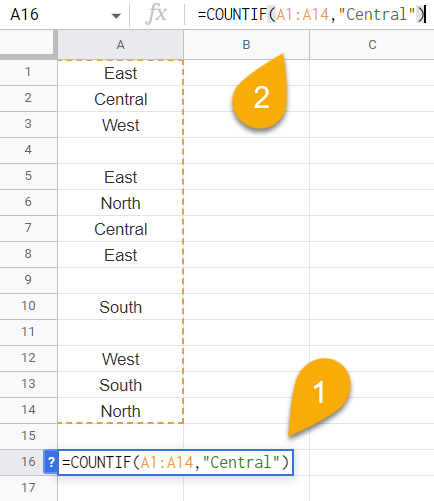1. Hit Enter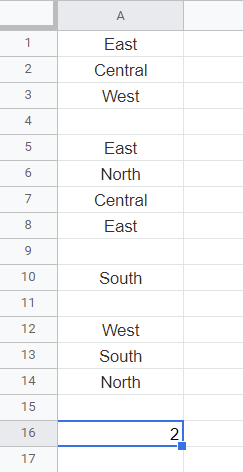Easy-peasy!

## How to Count Cells with Contents That Partially Match Specific Text

In order to do this, the following formula will come in handy.

Difficulty: Beginner

Time Estimate: 5 seconds

1. Choose the cell where you want the result.
2. In the Formula bar, type the formula =COUNTIF(A1:A14,”*e*”). Here A1:A14 is the cell range, and e is the specific text you need to find.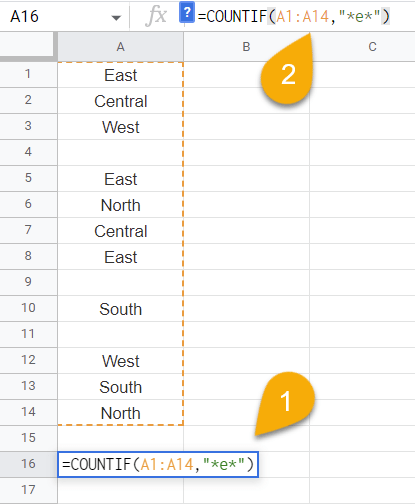1. Press the Enter key on your keyboard.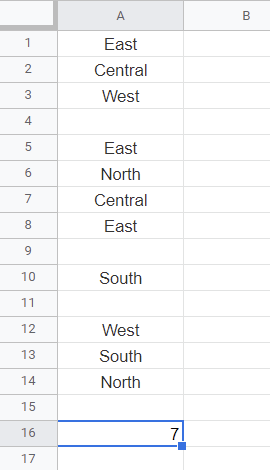That’s all there is to it!

## How to Count Cells with Specific Text Based on Multiple Conditions

If you need to apply multiple conditions in a formula, we recommend that you review the following step-by-step algorithm.

Difficulty: Beginner

Time Estimate: 10 seconds

1. Click on a blank cell.
2. Navigate to the Formula bar and type =ArrayFormula(SUM(COUNTIF(A1:A14,{“Central”, “West”}))A1:A14 is the cell range, and Central and West are words we need to find.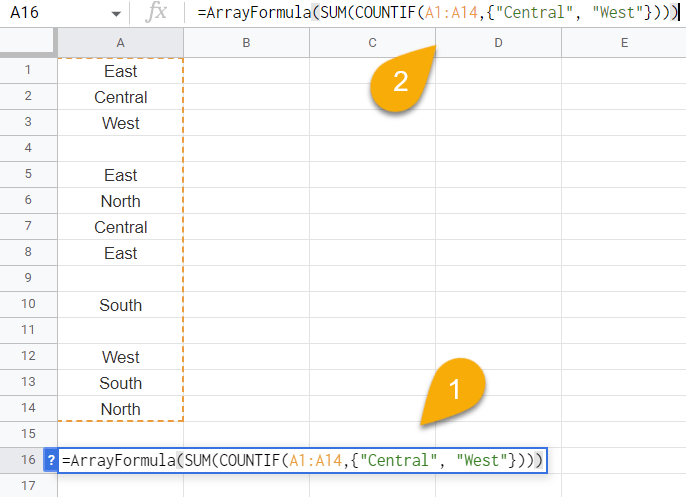1. Hit Enter.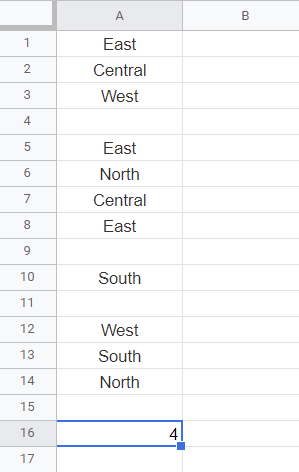And there’s the result! Feel free to modify the formulas as needed.